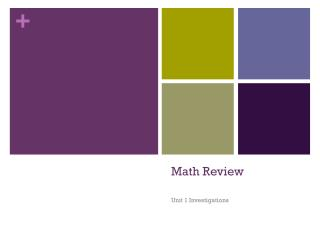DownloadDownload PresentationMath Review

# Math Review

Download Presentation## Math Review

- - - - - - - - - - - - - - - - - - - - - - - - - - - E N D - - - - - - - - - - - - - - - - - - - - - - - - - - -
##### Presentation Transcript

1. Math Review Unit 1 Investigations

2. Prime and Composite Numbers Prime Numbers: ONLY have two factors of one and itself. Practice Problem Question: Which of the following Numbers are prime? • 24 • 13 • 16 • 9

3. Prime and Composite Numbers • Composite Numbers: Have MORE than two factors. Practice Problem Question: Which of the following numbers is a composite number? • 7 • 5 • 30 • 19

4. Word Problems • Tip: Word problems do not have to be overwhelming. Take a deep breathe and visualize the problem in your mind. Don’t forget to reread the problem if you need to for comprehension. Let’s Practice Word Problems!!!!

5. Practice One Part A: Jordynalways takes the same route when she walks her dog. First, she walks 7 blocks to the park. Then she walks 9 blocks to the elementary school. How many blocks does Jordyn'sdog walk each day? Part B: If Jordyn decides to walk her dog twice a day for ten days, how many blocks will have Jordyn walked her dog?

6. Practice #2 In the magazine aisle at the grocery store there are 17 rows of magazines. In each row there are 13 magazines. How many magazines are in the aisle?

7. Practice #3 Rebecca babysat for 15 weeks over the summer. She earned \$75 each week. How much did Rebecca earn in all?

8. Challenge Problem!!! 8 ghouls each had 8 hands. Each hand had 8 warts and each wart had 8 hairs. How many hairs were there?

9. Spiral Review: Properties • Which of the following expressions would go in the  to demonstrate the associative property of multiplication? 12 × (8 × 14) = • (12 + 8) × 14 • (12 × 8) × 14 • (12 + 14) + 8 • 12 × (14 × 8)

10. Sprial Review: Properties Which property of whole numbers is demonstrated in the number sentence below? 16 + 25 = 25 + 16 Commutative property of addition Identity property of addition Commutative property of multiplication D. Associative property of addition

11. Spiral Review: Properties Which number sentence below demonstrates the identity property of multiplication? 16 × 18 = 18 × 16 (16 + 18) + 0 = 16 + 18 (16 × 18) × 1 = 16 × 18 (16 + 18) × 0 = 0

12. Spiral Review: Place Value Which number is greater than 415.043? 415.009 415.051 415.041 415.039

13. Spiral Review: Place Value Which statement below is true? 375,897 > 375,899 245,717 < 245,599 485,590 > 485,499 179,990 < 178,995

14. Spiral Review: Expanded Notation • Write in Standard Form: • 1. 2,000 + 400 + 30 + 5 = • 6,000 + 600 + 4 = • Write in Expanded Form: • 3.560.23 • 4. 1,212.45